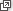## Assumed knowledge

• Basic skills for simplifying algebraic expressions and algebraic fractions.
• Expanding brackets.
• Factorising linear and quadratic expressions.
• Solving linear equations and inequalities.
• Solving quadratic equations by factoring, completing the square and applying the quadratic formula.
• Solving simultaneous linear equations.
• Substitution.

## Motivation

Algebra is the language of mathematics. Just as ordinary language is essential in verbal and written communication, so the ability to read, write and simplify algebraic expressions is fundamental to mathematical understanding, problem solving and the communication of mathematical ideas.

Students need to be able to write mathematics succinctly and correctly. The equal sign must only be placed between two quantities or expressions that are equal and never between two that are not. The solution to a problem should be able to be read so that each line follows from the previous line in a way that makes sense logically and symbolically. This is a skill that takes students some time and effort to master and it is the teacher's role here to act as a model par excellence and as a corrector of poorly written work.

A failure to write mathematics logically and correctly leads to and reflects a failure to think logically.

All of the algebra covered in this review is covered in much greater detail in the TIMES modules.

The relevant TIMES modules are:

Students and teachers should be giving some thought as to the logical flow when solving equations and writing algebra. When we write

$x-3 = 5, \quad x=8,$

we are really saying that each of these statements is logically equivalent to the other. We really mean, of course, that $$x-3=5$$ implies $$x=8$$ and $$x=8$$ implies $$x-3=5$$. This can be written formally as $$x-3=5 \iff x=8$$, but we do not use this sort of notation in school mathematics. On the other hand, when we write

$x=3, \quad\text{so } x^2=9,$

we need to be aware that the implication only goes one way. That is, the statement $$x^2=9$$ does not necessarily imply that $$x=3$$.

This module will provide a summary and review of this material. It is left up to the teacher to decide how much of this needs to be retaught and how much time should be spent on it. A degree of comfort and fluency with the content of this module is, however, essential before tackling calculus.

Next page - Content - Algebraic manipulation